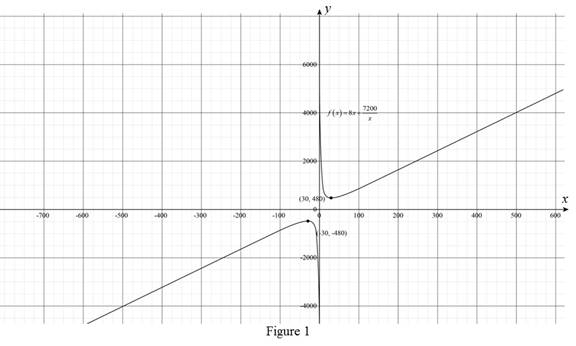# The function that models the cost of fencing of the garden.### Precalculus: Mathematics for Calcu...

6th Edition
Stewart + 5 others
Publisher: Cengage Learning
ISBN: 9780840068071### Precalculus: Mathematics for Calcu...

6th Edition
Stewart + 5 others
Publisher: Cengage Learning
ISBN: 9780840068071

#### Solutions

Chapter 2, Problem 23P

(a)

To determine

## To find: The function that models the cost of fencing of the garden.

Expert Solution

The function that models the cost of fencing of the garden is f(x)=8x+7200x.

### Explanation of Solution

Formula used:

The area of the rectangle is,

Perimeter of the rectangle is,

Given:

The cost of fence next to the road is $3 per foot and the cost of fence of other boundaries is$5 per foot.

The total area of the garden is 1200ft2.

Calculation:

Let the length of the rectangular garden is x units and the breadth of the rectangular garden is y units.

Substitute x for length and y for breadth in equation (1),

A=xy1200=xyy=1200x (3)

Now, substitute x for length and y for breadth in equation (2),

Then, the cost of fencing is,

f=5x+3y+3x+3y=8x+6y (4)

Substitute 1200x for y in equation (4),

f=8x+6y=8x+6(1200x)=8x+7200x

Thus, the function that models the cost of fence of the garden is f(x)=8x+7200x.

(b)

To determine

### To find: The garden dimensions that minimize the cost of fence.

Expert Solution

The length of the garden that minimize the cost of fence is 30ft and the breadth is 40ft.

### Explanation of Solution

Calculation:

To find the minimum cost of the fence of the rectangular garden, the graph of the function f(x)=8x+7200x has to be drawn.

The function contains the variable x as the length of the rectangular garden.

The local minimum value of the function is the least finite value where the value of the function at the any number is less than to the original function.

The condition for local minimum is,

f(a)f(x)

The graph of the function is shown below,From the above Figure, it can be observed that the least peak is at the point (30,480).

Then the minimum cost is $480 at x=30. Substitute x=30 in equation (3), y=120030=40 Thus, the length of the rectangular garden is 30 units and the breadth is 40 units. (c) To determine ### To find: The range of length that can be fenced along the road with an amount of$600.

Expert Solution

The range of the length of the rectangular garden that owner fence along the road is 15ftto 60ft.

### Explanation of Solution

Calculation:

From the part (a), cost function f(x)=8x+7200x.

If the owner has at most $600 then the cost function f(x)=8x+7200x is less than or equal to$600.

Substitute $600 for f(x) in cost function f(x)=8x+7200x, 8x+7200x600 (5) Take the equality of the equation (5), 8x+7200x=6008x2600x+7200=0x275x+900=0 Further solving, x260x15x+900=0x(x60)15(x60)=0(x60)(x15)=0x=60 and x=15 Thus, the range of the length is between 15ftto 60ft to fence the length of rectangular garden if the owner has at most$600.

### Have a homework question?

Subscribe to bartleby learn! Ask subject matter experts 30 homework questions each month. Plus, you’ll have access to millions of step-by-step textbook answers!